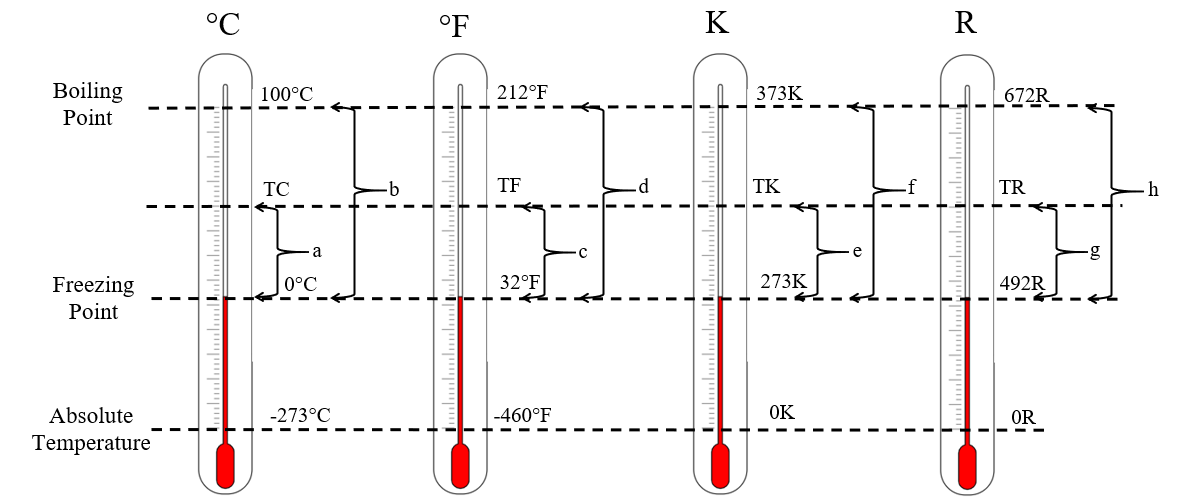# Problem of the day! (Thermodynamics)

in #ocd8 months ago

Derivation of the following temperatures formula:

Fahrenheit to Celsius

Celsius to Fahrenheit;

Fahrenheit to Kelvin

Kelvin to Fahrenheit

Celsius to Kelvin

Kelvin to Celsius

Fahrenheit to Rankine

Rankine to Fahrenheit

Celsius to Rankine

Rankine to Celsius

Solution:

Let;

Temperature in Celsius equal to TC

Temperature in Fahrenheit equal to TF

Temperature in Kelvin equal to TK

Temperature in Rankine equal to TR

So, based from the illustration below.using interpolation method;

TF to TC

a/b = c/d

where; a = TC – 0; b = 100 – 0; c = TF – 32; d = 212 – 32

(TC-0)/(100-0) = (TF-32)/(212-32)

TC/100 = (TF-32)/180

(TC)(180)/100 = TF -32

TF = [(TC)(180)/100] + 32

TF = 9/5(TC) + 32 (conversion from Fahrenheit to Celsius)

TC to TF

TF = 9/5(TC) + 32

9/5(TC) = TF – 32

**TC = 5/9(TF-32) (conversion from Celsius to Fahrenheit) **

TF to TK

c/d = e/f

where; c = TF – 32; d = 212 – 32; e = TK – 273; f = 373 – 273

(TF-32)/(212-32) = (TK-273)/(373-273)

(TF-32)/(180) = (TK-273)/(100)

TF-32 = (TK-273)(180)/100

TF-32 = 9/5(TK-273)

**TF = 9/5(TK-273) + 32 (conversion from Fahrenheit to Kelvin) **

TK to TF

TF = 9/5(TK-273) + 32

9/5(TK-273) = TF-32

TK-273 = 5/9(TF-32)

TK = 5/9(TF-32) + 273 (conversion from Kelvin to Fahrenheit)

TC to TK

a/b = e/f

where; a = TC – 0; b = 100 – 0; e = TK – 273; f = 373 – 273

(TC-0)/(100-0) = (TK-273)/(373-273)

TC/100 = (TK-273)/(100)

**TC = TK-273 (conversion from Celsius to Kelvin) **

TK to TC

TC = TK-273

TK = TC+273 (conversion from Kelvin to Celsius)

TF to TR

c/d = g/h

Where; c = TF – 32; d = 212 – 32; g = TR – 492; h = 672 – 492

(TF-32)/(212-32) = (TR-492)/(672-492)

(TF-32)/180 = (TR-492)/180

TF-32 = TR-492

TF = TR – 492 +32

**TF = TR – 460 (conversion from Fahrenheit to Rankine) **

TR to TF

TF = TR – 460

**TR = TF + 460 (conversion from Rankine to Fahrenheit) **

TC to TR

a/b = g/h

Where; a = TC – 0; b = 100 – 0; g = TR – 492; h = 672 – 492

(TC-0)/(100-0) = (TR-492)/(672-492)

TC/100 = (TR-492)/180

TC = (TR-492)(100)/180

**TC = 5/9(TR-492) (conversion from Celsius to Rankine) **

TR to TC

TC = 5/9(TR-492)

TC(9/5) = TR-492

TR = 9/5(TC) + 492 (conversion from Rankine to Celsius)